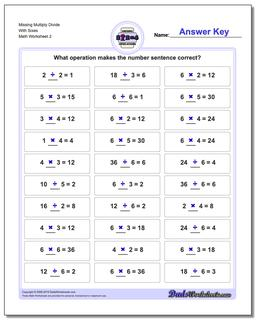# Math Worksheets: Missing Operations: Missing Operations: Missing Multiply Divide With Sixes (Second Worksheet)<worksheet-schema-block title='Missing Multiply Divide With Sixes (Second Worksheet)' description='Missing Multiply Divide With Sixes: Put your fingers away, boys and girls! If you are learning multiplication and division fact families, you need to memorize the facts, but if you don't even know where to start just understanding which result makes sense can be a good step. These missing operator worksheets for multiplication and division build basic number sense and will get you on the way multiplying and dividing like a professional. (Second Worksheet)' pagetype='Worksheet' alignment='2nd Grade, 3rd Grade, 4th Grade, 5th Grade' agerange='7-11'>